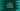# C program to calculate the area of a rectangle## Introduction :

This is a quick beginner level C programming question. We will learn how to calculate the area of a rectangle using user input values. Our program will ask the user to enter the height and width of the rectangle. It will calculate the area and print out the result.

With this program, you will learn how to read user inputs, how to do mathematical calculations and how to print values in C programming language.

### C program :

``````#include<stdio.h>

int main()
{

printf("Enter the length of the rectangle : ");
scanf("%f", &length);

printf("Enter the breadth of the rectangle : ");

printf("Area : %.2f\n", area);
}
``````

### Explanation :

1. length, breadth and area are three variables initialized to hold the length, breadth and area of the rectangle. These are all floating point variables.
2. Ask the user to enter the length of the rectangle. Read it and store it in variable length. We are using %f in scanf because this is a float value we are reading the user input.
4. Calculate the area by multiplying length and breadth.
5. Print the area variable to the user. Note that we are using %.2f to print it. This is because %.2f will put only two numbers to the right of the decimal point.

### Sample output :

``````Enter the length of the rectangle : 10
Enter the breadth of the rectangle : 10
Area : 100.00

Enter the length of the rectangle : 12
Enter the breadth of the rectangle : 8.5
Area : 102.00
``````